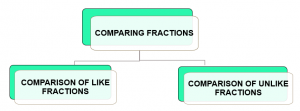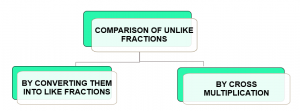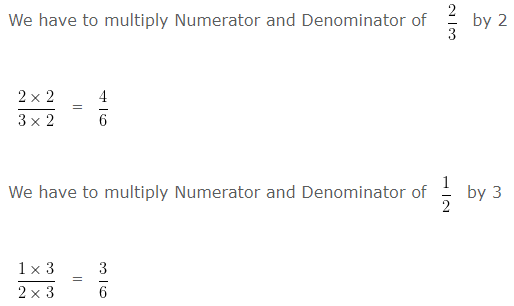# Comparing Fractions

Comparing Fractions can be divided in two categories:

• Comparison of Like Fractions (Comparing fractions with same denominators)
• Comparison of Unlike Fractions (Comparing fractions with different denominators)
First Method : By Converting Given Fractions into Like Fractions
Second Method : By Cross Multiplication Method### 1. Comparison of Like Fractions (Comparing fractions with same denominators)

QUESTION

Compare 7/3 and 4/3

EXPLANATION

7/3 and 4/3 are Like Fraction as Denominator of both the Fractions is same i.e, 3

In case of Like Fraction, the Fraction with the higher numerator is Higher

On Comparing the Numerators, we find

7 > 4

Hence, 7/3 > 4/3

### 2. Comparison of Unlike Fractions (Comparing fractions with different denominators)

QUESTION

Compare 2/3 and 1/2

EXPLANATION

2/3 and 1/2 are Unlike Fractions as Denominator of both the Fractions is different i.e, 3 and 2

To compare Unlike Fractions we have two methods:First Method : By Converting Given Fractions into Like Fractions

Given Fractions :

2/3 and 1/2

Taking LCM of the Denominator of both the Fractions.

i.e, LCM of 3 and 2 is 6

To change the Unlike Fractions into Like Fractions

We have to multiply the Numerator and Denominator of the Given Fraction by a number such that the Denominator of the given Fraction becomes equal to its LCM i.e, 6]

Now the Given Fractions are Like Fraction as their Denominator become same.

On Comparing their Numerators, we find

4 > 3

Therefore, 4/6 > 3/6

Hence, 2/3 > 1/2

Second Method : By Cross Multiplication Method

On Cross multiplying 2/3 and 1/2

In Cross Multiplication Method we multiply the Numerator of First Fraction namely 2 by the Denominator of Second Fraction namely 2 i.e,

2 x 2 = 4

Then, we multiply the Numerator of Second Fraction namely 1 by the Denominator of First Fraction namely 3 i.e,

1 x 3 = 3

Since, 4 > 3

Therefore,  2/3 > 1/2